HOME / Applications / Wilkinson RF Power divider

# Wilkinson RF Power divider

Used Tools:## Description

The Wilkinson power divider was for the first time introduced by Ernest Wilkinson in 1960. His proposed configuration was able to either split an input signal in two in-phase output signals or combine two equal phase input signals. The technique used to match the split ports to the common port is the quarter wave transformer. To match all three ports simultaneously, there is also a resistor between the second and third the port. This resistor basically isolates these two ports at the operating frequency of the splitter. In this example, a Printed Wilkinson RF Power Splitter operating at 9 GHz, has been modeled with HFWorks and the reflection and transmission coefficients along with other parameters are presented.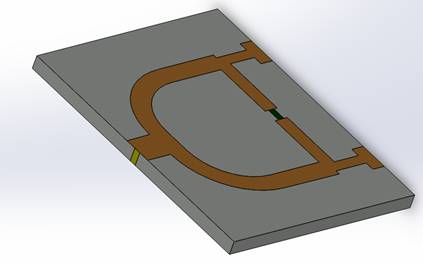Figure 1 - 3D view of the modeled Wilkinson divider

The model has been optimized: the best dimensions were used to draw the example in 3D. the dimensions are described within this figure.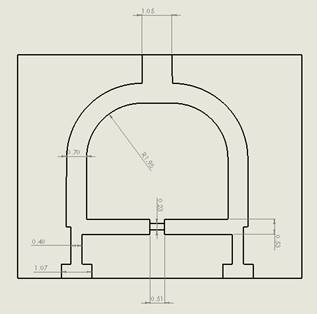## Simulation

The simulation of this power divider was run on an Scattering Parameters study from 4 GHz to 12 GHz. The circuit was proven efficient in equally splitting the power into two in-phase outputs.

## Solids and Materials

Taking into account the very narrow depth of the skin effect, we can model several conductors by assigning air to them. The material of the substrate is freely chosen from the library of the materials; We can apply materials that we define ourselves. Along with that material, we can specify a Signal boundary condition for the RF carrier and a PEC boundary condition for the ground metal beneath the substrate.

The ports are applied to the pad of the main excitation path. The conductor that carries the RF is assigned a signal boundary condition.

## Meshing

The model doesn't have very particular shapes. A uniform mesh of  the assembly should be sufficient without applying particular mesh controls.

## Results

Animating the 3D electric field's distribution by varying the omega-T angle gives us a hint on how the wave propagates into the port and gets 'splitted' within the two branches of the divider. We can first have a look at the curve of the reflection coefficient at the port, to decide which frequency yields the best matching and thus the best propagation into the two ports, but it is possible to view the near field even for frequencies where no propagation takes place.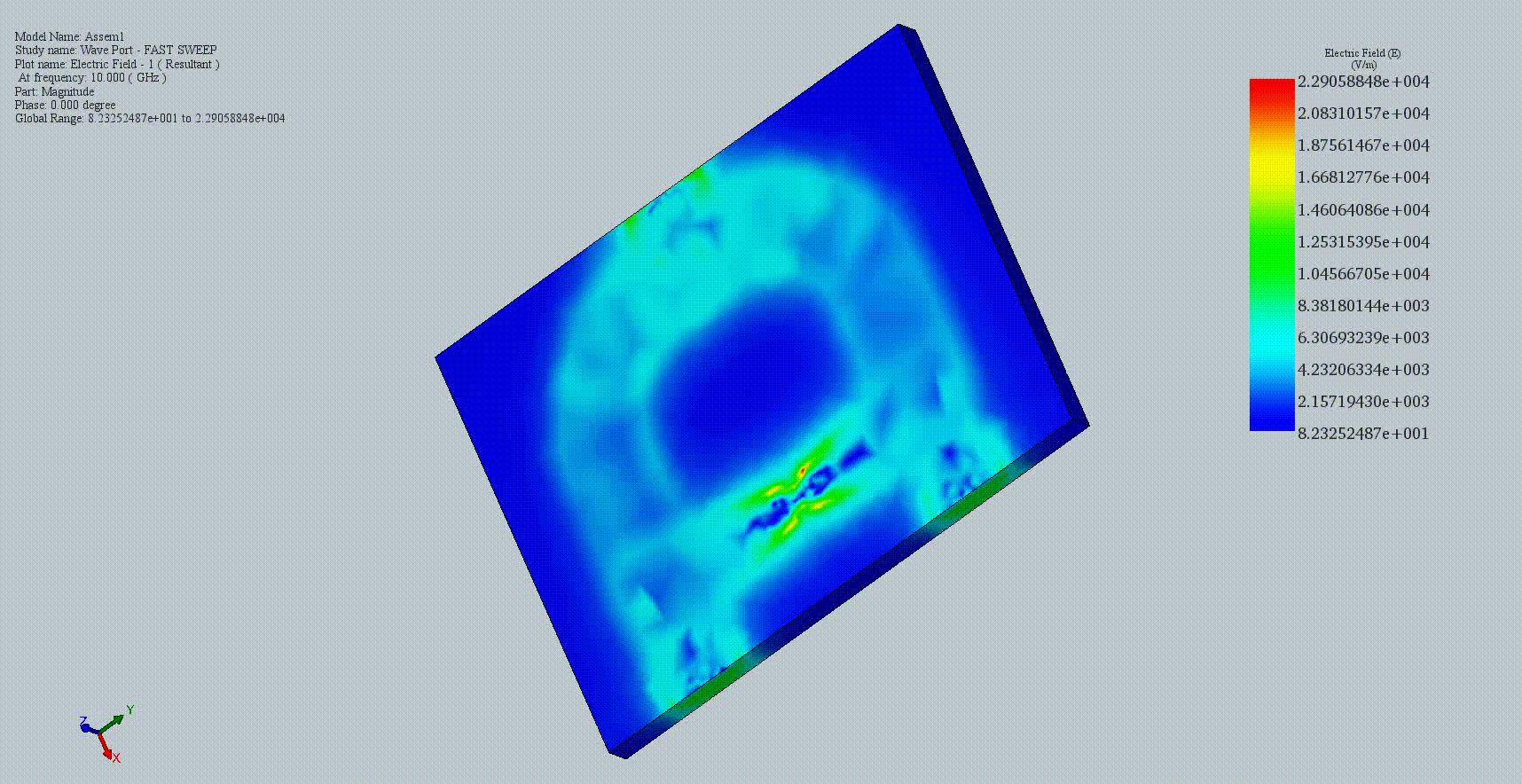Figure 3 - Wave propagation in the power divider at 10 GHz

This figure shows plots of the variation of the reflection coefficient and insertion loss of the divider.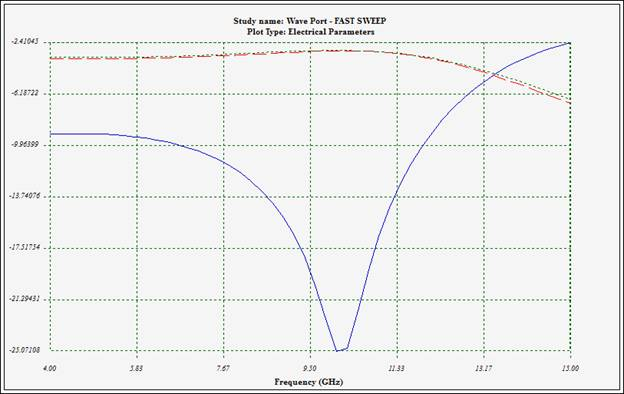Blue= S11; Red=S21; Green=S31

Figure 4 - Variations of reflection coefficient and insertion losses at the outputs

We can see that the two outputs present a 3 dB power loss relatively to the input: the power is well split into halves. In this figure, we show generalized Scattering Parameters matrix shown in dB/Phase format (polar with magnitude expressed in dB); we can see that S21 and S31 are almost the same (-3 dB=0.5) and both isolation and return loss are better than -14 dB.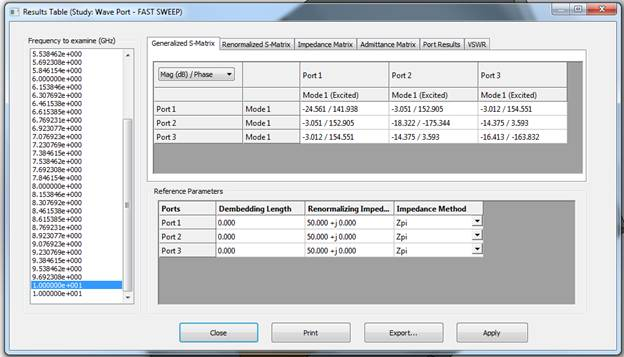Figure 5 - Results table with  various calculated parameters (within the tabs)